# MutuallyRecursive Data Definitions CS 5010 Program Design Paradigms

• Slides: 20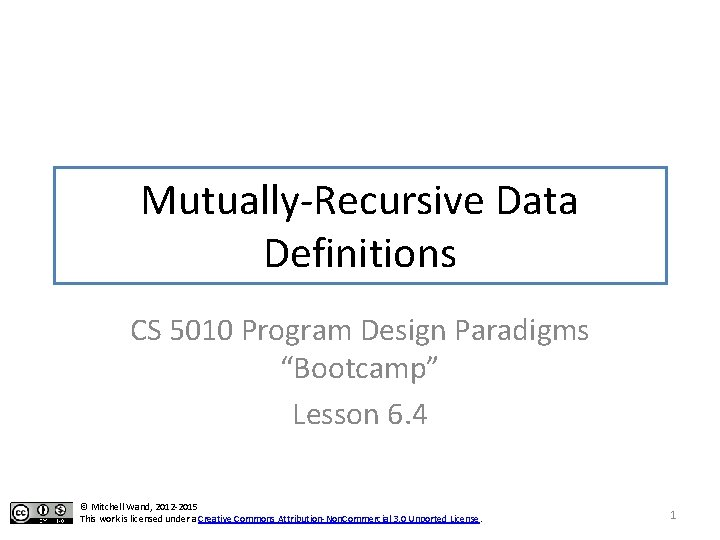Mutually Recursive Data Definitions • Sometimes two kinds of data are intertwined • In this lesson, we'll consider an easy example: alternating lists • An alternating list is a list whose elements alternate between numbers and strings 2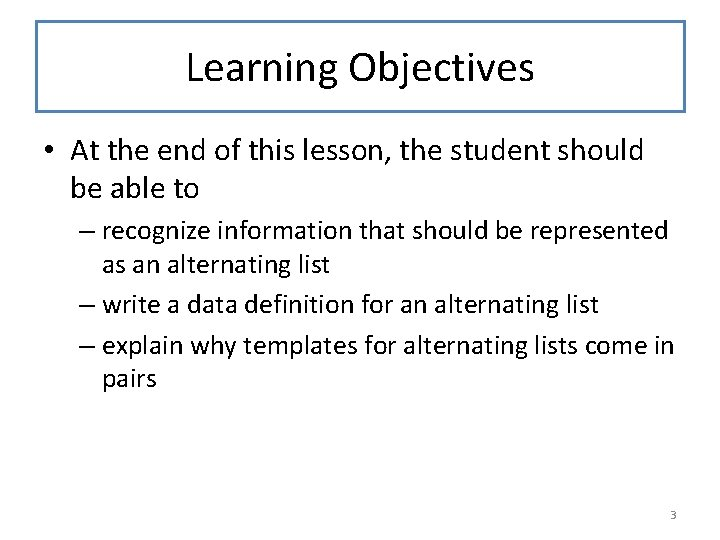Learning Objectives • At the end of this lesson, the student should be able to – recognize information that should be represented as an alternating list – write a data definition for an alternating list – explain why templates for alternating lists come in pairs 3Alternating Lists • Let's write a data definition for lists whose elements alternate between numbers and strings. 4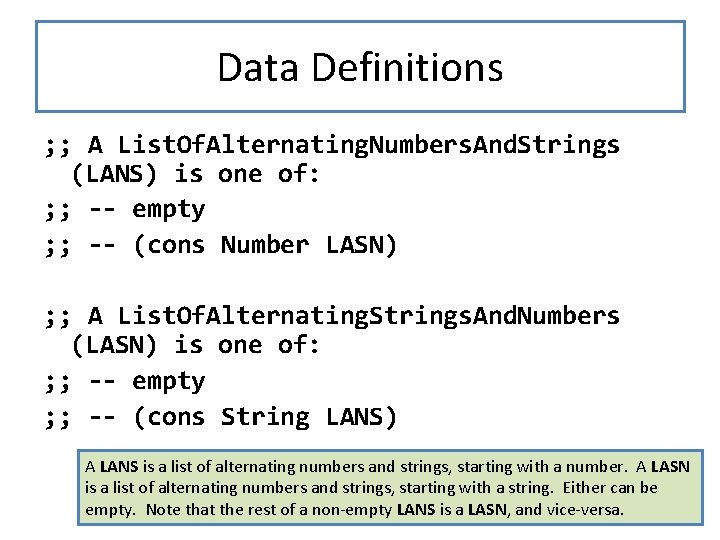Data Definitions ; ; A List. Of. Alternating. Numbers. And. Strings (LANS) is one of: ; ; -- empty ; ; -- (cons Number LASN) ; ; A List. Of. Alternating. Strings. And. Numbers (LASN) is one of: ; ; -- empty ; ; -- (cons String LANS) A LANS is a list of alternating numbers and strings, starting with a number. A LASN is a list of alternating numbers and strings, starting with a string. Either can be empty. Note that the rest of a non-empty LANS is a LASN, and vice-versa. 5Examples (cons "foo" (cons 23 (cons "foo" (cons "bar" (cons 23 (cons "foo" (cons 11 11 empty))) empty)))) is is is a a a LASN LANS LASN 6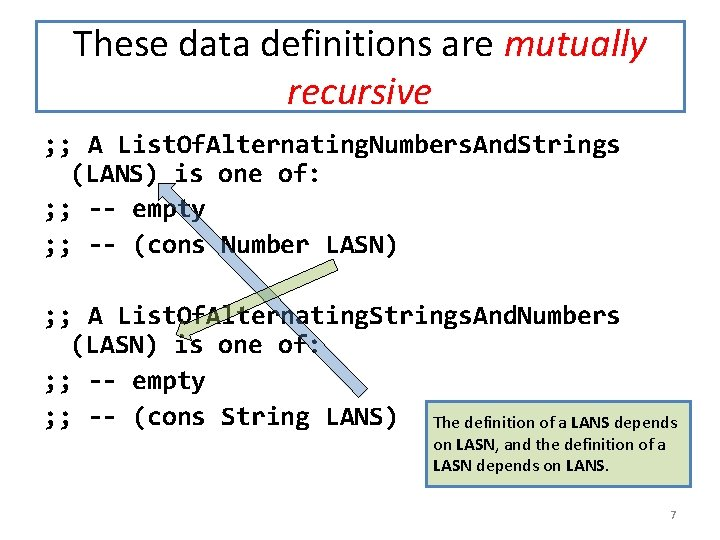These data definitions are mutually recursive ; ; A List. Of. Alternating. Numbers. And. Strings (LANS) is one of: ; ; -- empty ; ; -- (cons Number LASN) ; ; A List. Of. Alternating. Strings. And. Numbers (LASN) is one of: ; ; -- empty ; ; -- (cons String LANS) The definition of a LANS depends on LASN, and the definition of a LASN depends on LANS. 7This is mutual recursion defined in terms of LASN LANS defined in terms of 8The template recipe Question Answer Does the data definition distinguish among different subclasses of data? Your template needs as many cond clauses as subclasses that the data definition distinguishes. How do the subclasses differ from each other? Use the differences to formulate a condition per clause. Do any of the clauses deal with structured If so, add appropriate selector expressions values? to the clause. Does the data definition use selfreferences? Formulate ``natural recursions'' for the template to represent the self-references of the data definition. Do any of the fields contain compound or mixed data? If the value of a field is a foo, add a call to a foo-fn to use it. The template recipe doesn't need to change 9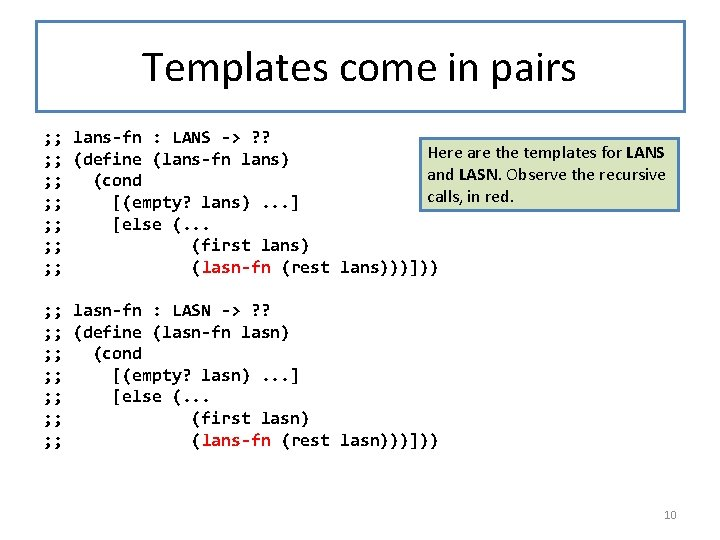Templates come in pairs ; ; lans-fn : LANS -> ? ? Here are the templates for LANS ; ; (define (lans-fn lans) and LASN. Observe the recursive ; ; (cond calls, in red. ; ; [(empty? lans). . . ] ; ; [else (. . . ; ; (first lans) ; ; (lasn-fn (rest lans)))])) ; ; lasn-fn : LASN -> ? ? ; ; (define (lasn-fn lasn) ; ; (cond ; ; [(empty? lasn). . . ] ; ; [else (. . . ; ; (first lasn) ; ; (lans-fn (rest lasn)))])) 10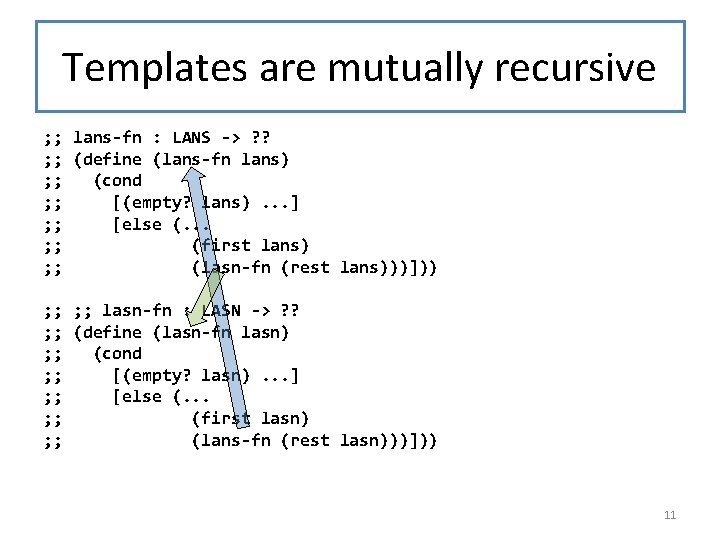Templates are mutually recursive ; ; lans-fn : LANS -> ? ? ; ; (define (lans-fn lans) ; ; (cond ; ; [(empty? lans). . . ] ; ; [else (. . . ; ; (first lans) ; ; (lasn-fn (rest lans)))])) ; ; lasn-fn : LASN -> ? ? ; ; (define (lasn-fn lasn) ; ; (cond ; ; [(empty? lasn). . . ] ; ; [else (. . . ; ; (first lasn) ; ; (lans-fn (rest lasn)))])) 11This is mutual recursion defined in terms of lasn-fn lans-fn defined in terms of Here's that same picture, this time describing the recursive calls in the template. 12The template questions What is the answer for the ; ; lans-fn : LANS -> ? ? empty LANS? ; ; (define (lans-fn lans) ; ; (cond ; ; [(empty? lans). . . ] ; ; [else (. . . If you knew the answer for the ; ; (first lans) LASN inside the LANS, what ; ; (lasn-fn (rest lans)))])) would the answer be for the whole LANS? ; ; lasn-fn : LASN -> ? ? ; ; (define (lasn-fn lasn) What is the answer for the ; ; (cond empty LASN? ; ; [(empty? lasn). . . ] ; ; [else (. . . ; ; (first lasn) If you knew the answer for the ; ; (lans-fn (rest lasn)))])) LANS inside the LASN, what As usual, we have one would the answer be for the question for each blank whole LASN? 13 in the template.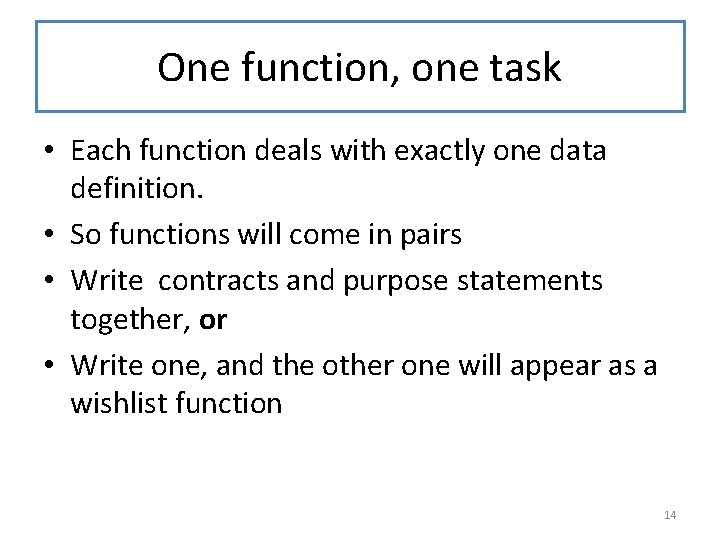One function, one task • Each function deals with exactly one data definition. • So functions will come in pairs • Write contracts and purpose statements together, or • Write one, and the other one will appear as a wishlist function 14Example lans-sum : LANS -> Number Returns the sum of all the numbers in the given Lans lasn-sum : LASN -> Number Returns the sum of all the numbers in the given Lasn Here's an example of a pair of functions that should go together. 15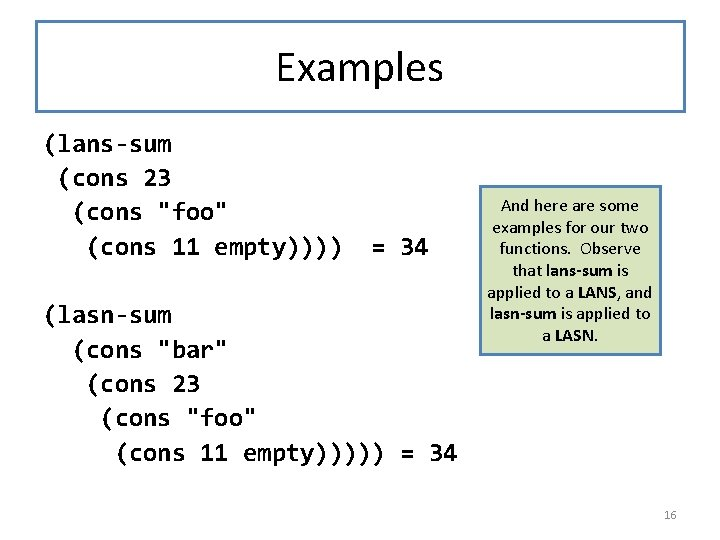Examples (lans-sum (cons 23 (cons "foo" (cons 11 empty)))) = 34 (lasn-sum (cons "bar" (cons 23 (cons "foo" (cons 11 empty))))) = 34 And here are some examples for our two functions. Observe that lans-sum is applied to a LANS, and lasn-sum is applied to a LASN. 16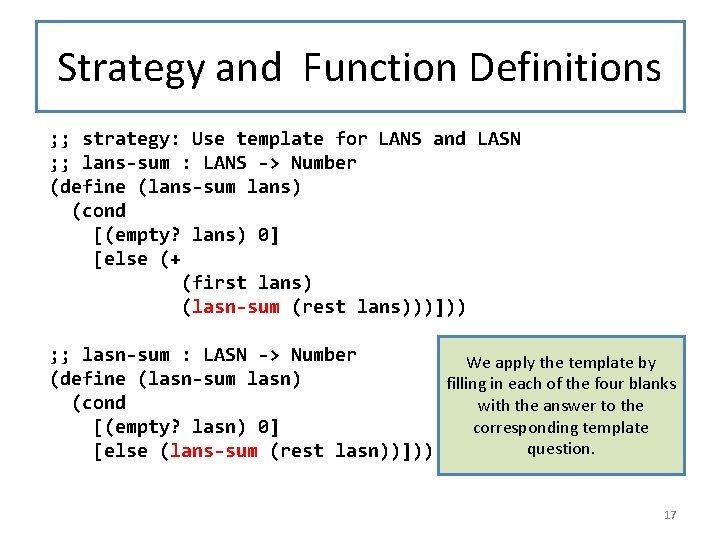Strategy and Function Definitions ; ; strategy: Use template for LANS and LASN ; ; lans-sum : LANS -> Number (define (lans-sum lans) (cond [(empty? lans) 0] [else (+ (first lans) (lasn-sum (rest lans)))])) ; ; lasn-sum : LASN -> Number We apply the template by (define (lasn-sum lasn) filling in each of the four blanks (cond with the answer to the corresponding template [(empty? lasn) 0] question. [else (lans-sum (rest lasn))])) 17What are alternating lists good for? ? Information ? ? ? representation interpretation Answer: Not much! Don't use them! Alternating Lists But they make a good example of mutuallyrecursive data definitions 18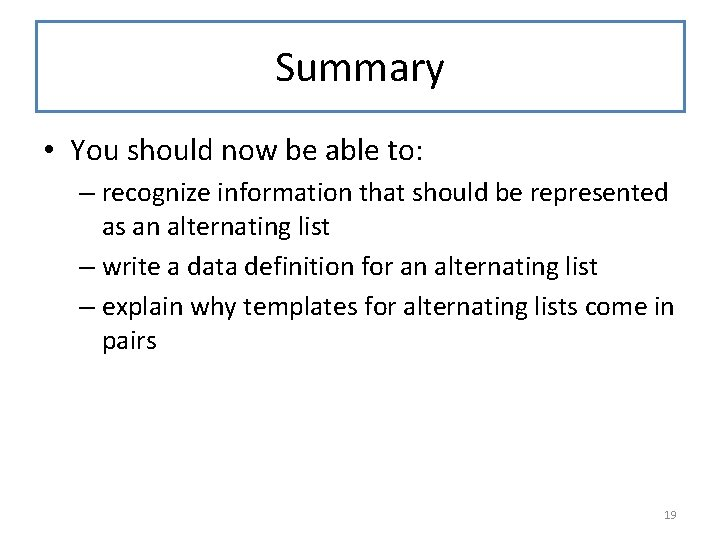Summary • You should now be able to: – recognize information that should be represented as an alternating list – write a data definition for an alternating list – explain why templates for alternating lists come in pairs 19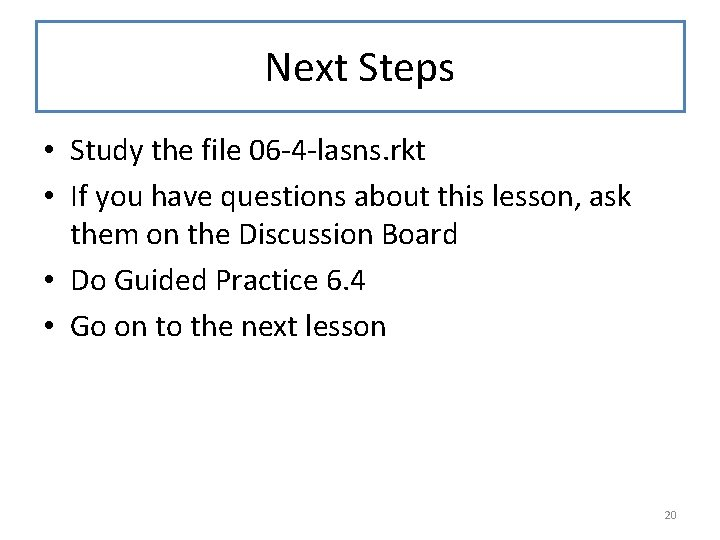Next Steps • Study the file 06 -4 -lasns. rkt • If you have questions about this lesson, ask them on the Discussion Board • Do Guided Practice 6. 4 • Go on to the next lesson 20